# Safe Flexible Hypothesis Tests for Practical Scenarios

Safe tests is a collective name for a new form of hypothesis tests that yield e-values (instead of p-values). The original paper on e-values by Grünwald, de Heide and Koolen can be found here. For each hypothesis testing setting where one would normally use a p-value, a safe test can be designed, with a number of advantages that are elaborately described and illustrated in this vignette. Currently, this package provide e-values for the t-test, Fisher’s exact test, and the chi-squared test (the safe test of 2 proportions). These safe tests were designed to be GROW; they perform the best under the worst case in the alternative hypothesis (see the original paper).

Technically, E-values are non-negative random variables (test statistics) that have an expected value of at most one under the null hypothesis. The E-value can be interpreted as an gamble against the null hypothesis in which an investment of 1$returns E$ whenever the null hypothesis fails to hold true. Hence, the larger the observed e-value, the larger the incentive to reject the null.

A big advantage of the e-values over their p-value equivalents is that safe tests conserve the type I error guarantee (false positive rate) regardless of the sample size. This implies that the evidence can be monitored as the observations come in, and the researcher is allowed to stop the experiment early (optional stopping), whenever the evidence is compelling. By stopping early fewer participants will be put at risk. In particular, those patients who are assigned to the control condition, when a treatment is effective. Safe tests also allow for optional continuation, which means that the researcher can extend the experiment irrespectively of the motivation. For instance, if more funds become available, or if the evidence looks promising and the funding agency, a reviewer, or an editor urges the experimenter to collect more data.

Importantly, for the safe tests presented here neither optional stopping nor continuation leads to the test exceeding the promised type I error guarantee. As the results do not depend on the planned, current, or future sample sizes, safe tests allow for anytime valid inferences. We illustrate these properties below.

Firstly, we show how to design an experiment based on safe tests.

Secondly, simulations are run to show that safe tests indeed conserve the type I error guarantee under optional stopping. We also show that optional stopping causes the false null rejection rate of the classical p-value test to exceed the promised level \alpha type I error guarantee. This implies that with classical tests one cannot adapt to the information acquired during the study without increasing the risk of making a false discovery.

Lastly, it is shown that optionally continuing non-significant experiments also causes the p-value tests to exceed the promised level \alpha type I error guarantee, whereas this is not the case for safe tests.

This demonstration further emphasises the rigidity of experimental designs when inference is based on a classical test: the experiment cannot be stopped early, or extended. Thus, the planned sample size has to be final. As such, the protocol needs to account for possible future sample sizes, which is practically impossible to plan for. Even if such a protocol can be made, there is no guarantee that the experiments go exactly according to plan, as things might go wrong during the study.

The ability to act on information that accumulates during the study – without sacrificing the correctness of the resulting inference – was the main motivation for the development of safe tests, as it provides experimenters with the much needed flexibility.

## Installation

The stable version can be installed by entering in R:

install.packages("safestats")

The development version can be found on GitHub, which can be installed with the devtools package from CRAN by entering in R:

devtools::install_github("AlexanderLyNL/safestats", ref = "logrank", build_vignettes = TRUE)

The command

library(safestats)

# Test of Means: T-Tests

## 1. Designing Safe Experiments

### Type I error and type II errors

To avoid bringing an ineffective medicine to the market, experiments need to be conducted in which the null hypothesis of no effect is tested. Here we show how flexible experiments based on safe tests can be designed.

As the problem is statistical in nature, due to variability between patients, we cannot guarantee that 0% of the medicine that pass the test will be ineffective. Instead, the target is to bound the type I error rate by a tolerable \alpha, say, \alpha = 0.05. In other words, at most 5 out of the 100 inneffective drugs are allowed to pass the safe test.

At the same time, we would like to avoid a type II error, that is, missing out on finding an effect, while there is one. Typically, the targetted type II error rate is \beta = 0.20, which implies that whenever there truly is an effect, an experiment needs to be designed in such a way that with 1 – \beta = 80% chance the effect is detected.

### Case (I): Designing experiments with the minimal clinically relevant effect size known

Not all effects are equally important, especially, when a minimal clinically relevant effect size can be formulated. For instance, suppose that a population of interest has a population average systolic blood pressure of \mu = 120 mmHg and that the population standard deviation is \sigma = 15. Suppose further that all approved blood pressure drugs change the blood pressure by at least 9 mmHg, then a minimal clinically relevant effect size can be defined as \delta_{\min} = (\mu_{\text{post}} – \mu_{\text{pre}}) / (\sqrt{2} \sigma) = 9 / (15 \sqrt{2} ) = 0.42, where \mu_{\text{post}} represents the average blood pressure after treatment and \mu_{\text{pre}} the average blood pressure before treatment of the population of interest. The \sqrt{2}-term in the denominator is a result of the measurements being paired.

Based on a tolerable type I error rate of \alpha = 0.05, type II error rate of \beta = 0.20, and minimal clinical effect size of \delta_{\min} \approx 0.42, the following code shows that we then need to plan an experiment consisting of 63 patients each measured before (n2Plan) and after (n1Plan) the treatment.

alpha <- 0.05
beta <- 0.2
deltaMin <- 9/(sqrt(2)*15)

designObj <- designSafeT(deltaMin=deltaMin, alpha=alpha, beta=beta,
alternative="greater", testType="paired")
designObj
#>
#>  Safe Paired Sample T-Test Design
#>
#>                   n1Plan, n2Plan = 63, 63
#> minimal standardised effect size = 0.4242641
#>                      alternative = greater
#>                  power: 1 - beta = 0.8
#>                parameter: deltaS = 0.29
#>                            alpha = 0.05
#> decision rule: e-value > 1/alpha = 20
#>
#> Timestamp: 2020-11-02 15:44:36 CET

### Case (II): Minimal clinically relevant effect size unknown, but maximum number of samples known.

It is not always clear what the minimal clinically relevant effect size is. In that case, the design function can be called for a reasonable range of minimal clinically relevant effect sizes, when it is provided with the tolerable type I and type II error rates. Furthermore, when it is a priori known that only, say, 100 samples can be collected due to budget constraints, then the following function allows for a futility analysis:

# Recall:
# alpha <- 0.05
# beta <- 0.2

result <- plotSafeTDesignSampleSizeProfile(alpha=alpha, beta=beta,
maxN=100,
testType="paired")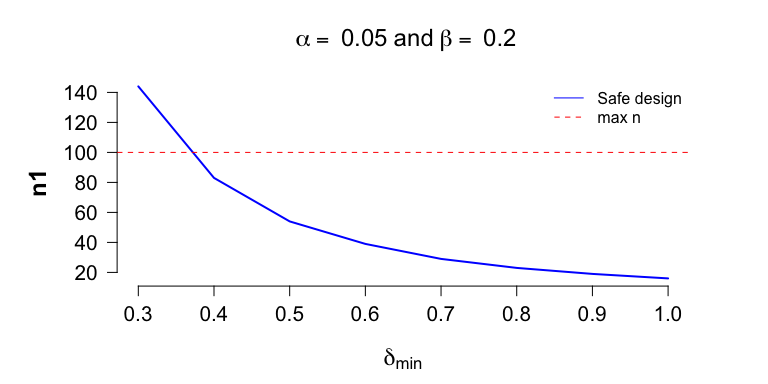The plot shows that when we have budget for at most 100 paired samples, we can only guarantee a power of 80%, if the true effect size is at least 0.37. If a field expert believes that an effect size of 0.3 is realistic, then the plot shows that we should either apply for additional grant money to test an additional 44 patients, or decide that it’s futile to set up this experiment, and spend our time and efforts on a different endeavour.

## 2. Inference with Safe Tests: Full experiment

Firstly, we show that inference based on safe tests conserve the tolerable \alpha-level, if the null hypothesis of no effect is rejected whenever the e-value, the outcome of a safe test, is larger than 1/\alpha. For instance, for \alpha = 0.05 the safe test rejects the null whenever the e-value is than 20. The level \alpha type I error rate is also guaranteed under (early) optional stopping. Secondly, we show that there is a high chance of stopping early whenever the true effect size is at least as large as the minimal clinically relevant effect size.

### Safe tests conserve the type I error rate: Full experiment

To see that safe tests only lead to a false null rejection very infrequently, we consider an experiment with the same number of samples as it was planned for, but with no effect, that is,

set.seed(1)
preData <- rnorm(n=designObj$nPlan, mean=120, sd=15) postData <- rnorm(n=designObj$nPlan, mean=120, sd=15)
# Thus, the true delta is 0:
# deltaTrue <- (120-120)/(sqrt(2)*15)     

The safe test applied to data under the null results in an e-value that is larger than 1/\alpha = 20 with at most \alpha = 5% chance. In particular,

safeTTest(x=preData, y=postData, alternative = "greater",
designObj=designObj, paired=TRUE)
#>
#>  Safe Paired Sample T-Test
#>
#> data:  preData and postData. n1 = 63, n2 = 63
#> estimates: mean of the differences = 1.1423
#>
#> test: t = 0.48905, deltaS = 0.29
#> e-value = 0.21959 > 1/alpha = 20 : FALSE
#> alternative hypothesis: true difference in means ('x' minus 'y') is greater than mu
#>
#> design: the test was designed with alpha = 0.05
#> for experiments with n1Plan = 63, n2Plan = 63
#> to guarantee a power = 0.8 (beta = 0.2)
#> for minimal relevant standardised effect size = 0.42426 (greater)

or equivalently with syntax closely resembling the standard t.test code in R:

safe.t.test(x=preData, y=postData, alternative = "greater",
designObj=designObj, paired=TRUE)
#>
#>  Safe Paired Sample T-Test
#>
#> data:  preData and postData. n1 = 63, n2 = 63
#> estimates: mean of the differences = 1.1423
#>
#> test: t = 0.48905, deltaS = 0.29
#> e-value = 0.21959 > 1/alpha = 20 : FALSE
#> alternative hypothesis: true difference in means ('x' minus 'y') is greater than mu
#>
#> design: the test was designed with alpha = 0.05
#> for experiments with n1Plan = 63, n2Plan = 63
#> to guarantee a power = 0.8 (beta = 0.2)
#> for minimal relevant standardised effect size = 0.42426 (greater)

The following code replicates this setting a 1,000 times and shows that indeed, only a very few times will the e-values cross the boundary of 1/\alpha under the null:

# alpha <- 0.05

set.seed(1)
eValues <- replicate(n=1000, expr={
preData <- rnorm(n=designObj$nPlan, mean=120, sd=15) postData <- rnorm(n=designObj$nPlan, mean=120, sd=15)
safeTTest(x=preData, y=postData, alternative = "greater",
designObj=designObj,paired=TRUE)$eValue} ) mean(eValues > 20) #>  0.008 mean(eValues > 20) < alpha #>  TRUE ### The designed safe tests is as powerful as planned: Full experiment If the true effect size equals the minimal clinical effect size and the experiment is run as planned, then the safe tests detects the effect with 1 – \beta = 80% chance as promised. This is shown by the following code for one experiment set.seed(1) preData <- rnorm(n=designObj$nPlan, mean=120, sd=15)
postData <- rnorm(n=designObj$nPlan, mean=111, sd=15) safeTTest(x=preData, y=postData, alternative = "greater", designObj=designObj, paired=TRUE) #> #> Safe Paired Sample T-Test #> #> data: preData and postData. n1 = 63, n2 = 63 #> estimates: mean of the differences = 10.142 #> #> test: t = 4.3424, deltaS = 0.29 #> e-value = 635.04 > 1/alpha = 20 : TRUE #> alternative hypothesis: true difference in means ('x' minus 'y') is greater than mu #> #> design: the test was designed with alpha = 0.05 #> for experiments with n1Plan = 63, n2Plan = 63 #> to guarantee a power = 0.8 (beta = 0.2) #> for minimal relevant standardised effect size = 0.42426 (greater) and by the following code for multiple experiments # Recall: # alpha <- 0.05 # beta <- 0.2 power <- 1-beta set.seed(1) eValues <- replicate(n=1000, expr={ preData <- rnorm(n=designObj$nPlan, mean=120, sd=15)
postData <- rnorm(n=designObj$nPlan, mean=111, sd=15) safeTTest(x=preData, y=postData, alternative = "greater", designObj=designObj, paired=TRUE)$eValue})
mean(eValues > 1/alpha)
#>  0.808
mean(eValues > 1/alpha) >= power
#>  TRUE

Due to sampling error, the average number of times that S > 1 / \alpha might not always be larger than the specified power, but it should always be close to it. The sampling error decreases as the number of replications increases and converges to 80%.

## Safe Tests Allow for Optional Stopping without Inflating the Type I Error Rate above the Tolerable \alpha-Level

What makes the safe tests in this package particularly interesting is that they allow for early stopping without the test exceeding the tolerable type I error rate of \alpha. This means that the evidence can be monitored as the data comes in, and when there is a sufficient amount of evidence against the null, thus, S > 1/\alpha, the experiment can be stopped early, which therefore increases efficiency.

Note that not all e-values necessarily allow for optional stopping: this only holds for some special e-values, that are also test martingales. More information can be found, for example, in the first author’s master thesis, Chapter 5.

For this purpose, we use the design that was derived above, that is,

designObj
#>
#>  Safe Paired Sample T-Test Design
#>
#>                   n1Plan, n2Plan = 63, 63
#> minimal standardised effect size = 0.4242641
#>                      alternative = greater
#>                  power: 1 - beta = 0.8
#>                parameter: deltaS = 0.29
#>                            alpha = 0.05
#> decision rule: e-value > 1/alpha = 20
#>
#> Timestamp: 2020-11-02 15:44:36 CET

### Safe tests detect the effect early if it is present: deltaTrue equal to deltaMin

The following code replicates 1,000 experiments and each data set is generated with a true effect size that equals the minimal clinical-relevant effect size of \delta_{\min}=9/(15 \sqrt{2}) = 0.42. The safe test is applied to each data set sequentially and if the e-value is larger than 1 / \alpha, the experiment is stopped. If the e-value does not exceed 1 / \alpha, the experiment is run until all samples are collected as planned.

# Recall:
# alpha <- 0.05
# beta <- 0.2
# deltaMin <- 9/(sqrt(2)*15)      # = 0.42
simResultDeltaTrueIsDeltaMin <- simulate(object=designObj, nsim=1000L,
seed=1, deltaTrue=deltaMin,
muGlobal=120, sigmaTrue=15)
#> ================================================================================
simResultDeltaTrueIsDeltaMin
#>
#>    Simulations for Safe Paired Sample T-Test
#>
#> Based on nsim = 1000 and if the true effect size is
#>     deltaTrue = 0.4242641
#> then the safe test optimised to detect an effect size of at least:
#>     deltaMin = 0.4242641
#> with tolerable type I error rate of
#>     alpha = 0.05 and power: 1-beta = 0.8
#> For experiments with planned sample sizes:
#>     n1Plan = 63 and n2Plan = 63
#>
#> Is estimated to have a null rejection rate of
#>     powerAtNPlan = 0.795
#> at the planned sample sizes.
#>
#> Is estimated to have a null rejection rate of
#>     powerOptioStop = 0.855
#> under optional stopping, and the average stopping time is:
#>     n1Mean = 39.494

The simulations show that the tolerable type II error rate of \beta = 0.2, which the experiments were planned for is almost reached, as 1 – 0.795 = 0.205. The discrepancy of 0.5% is due to sampling error and vanishes as the number of simulations increases. Note that optional stopping increases power to larger than the targetted 1 – \beta = 80%: the simulations demonstrate how power is gained as a result of optional stopping whenever the true effect size equals the minimal clinically relevant effect size.

Furthermore, the average sample at which the experiment is stopped is much lower than what was planned for. To see the distributions of stopping times, the following code can be run

plot(simResultDeltaTrueIsDeltaMin)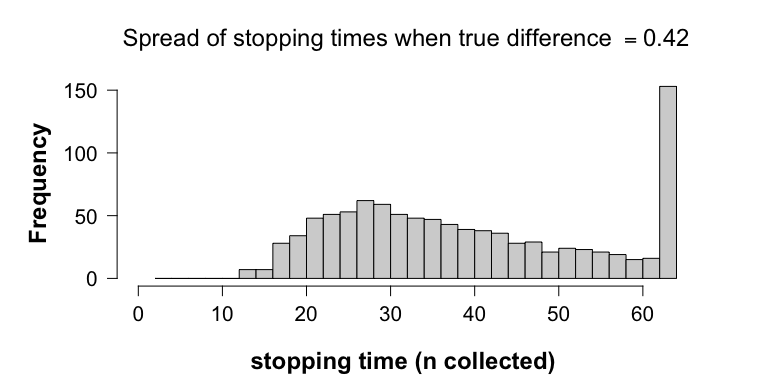The histogram shows that about 43 experiments (out of a 1,000) were stopped, at n1=n2=21 and n1=n2=22. These null rejections are correct and detected early on. The last bar collects all experiments that ran until the planned sample sizes, thus, also those that did not lead to a null rejection at n=63. To see the distributions of stopping times of only the experiments where the null is rejected, we run the following code:

plot(simResultDeltaTrueIsDeltaMin, showOnlyNRejected=TRUE)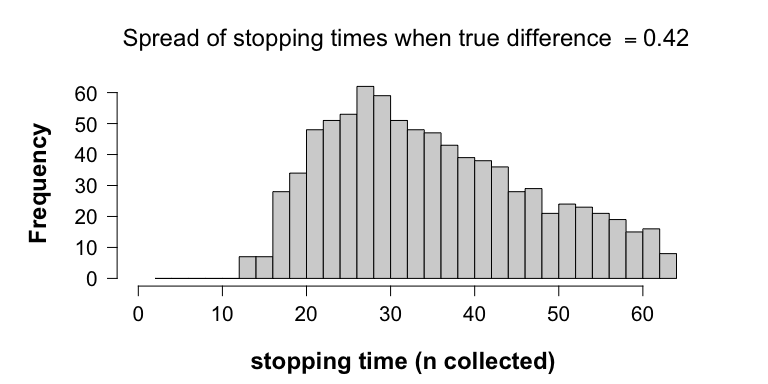### Safe tests detect the effect early if it is present: deltaTrue larger than deltaMin

What we believe is clinically minimally relevant might not match reality. One advantage of safe tests is that they perform even better, if the true effect size is larger than the minimal clinical effect size that is used in the planning of the experiment. To see this, we run the following code

# Recall:
# alpha <- 0.05
# beta <- 0.2
# deltaMin <- 9/(sqrt(2)*15)      # = 0.42
deltaTrueLarger <- 0.6

simResultDeltaTrueLargerThanDeltaMin <- simulate(object=designObj,
nsim=1000L, seed=1,
deltaTrue=deltaTrueLarger,
muGlobal=120, sigmaTrue=15)
#> ================================================================================
simResultDeltaTrueLargerThanDeltaMin
#>
#>    Simulations for Safe Paired Sample T-Test
#>
#> Based on nsim = 1000 and if the true effect size is
#>     deltaTrue = 0.6
#> then the safe test optimised to detect an effect size of at least:
#>     deltaMin = 0.4242641
#> with tolerable type I error rate of
#>     alpha = 0.05 and power: 1-beta = 0.8
#> For experiments with planned sample sizes:
#>     n1Plan = 63 and n2Plan = 63
#>
#> Is estimated to have a null rejection rate of
#>     powerAtNPlan = 0.979
#> at the planned sample sizes.
#>
#> Is estimated to have a null rejection rate of
#>     powerOptioStop = 0.992
#> under optional stopping, and the average stopping time is:
#>     n1Mean = 27.943

With a larger true effect size, the power at the sampled sample sizes increases from 79.5% to 97.9%. More importantly, this increase is picked up earlier by the designed safe test, and optional stopping allows us to act on this. Note that the average stopping time is now further decreased, from 39.494 to 27.943. This is apparent from the fact that the histogram of stopping times is now shifted to the left:

plot(simResultDeltaTrueLargerThanDeltaMin)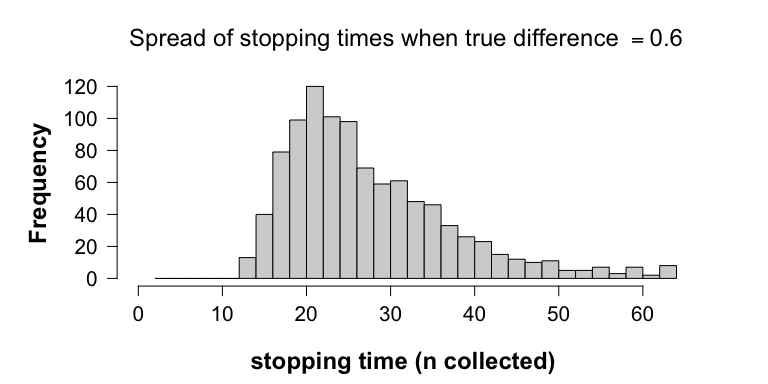Hence, this means that if the true effect is larger than what was planned for, the safe test will detect this larger effect earlier on, which results in a further increase of efficiency.

### Optional stopping does not causes safe tests to overreject the null, but is problematic for p-values

The previous examples highlight how optional stopping results in an increase in power, i.e., the chance of rejecting the null is increased, when the alternative is true. When the null holds true, however, the rejection rate should be low, at least not larger than the tolerable type I error rate. Here we show that optional stopping results in the type I error rate of the safe test to not exceed \alpha, whereas early stopping with classical p-value tests does result in the exceedance of the prescribed \alpha-level. In other words, optional stopping with p-values leads to an increased risk of falsely claiming that a medicine is effective, while in reality the effect is absent.

For this purpose we run the code

# Recall:
# alpha <- 0.05
# beta <- 0.2
# deltaMin <- 9/(sqrt(2)*15)      # = 0.42

freqDesignObj <- designFreqT(deltaMin=deltaMin, alpha=alpha, beta=beta,
alternative="greater", testType="paired")

simResultDeltaTrueIsZero <- simulate(object=designObj, nsim=1000L, seed=1,
deltaTrue=0, freqOptioStop=TRUE,
nPlanFreq=freqDesignObj$nPlan, muGlobal=120, sigmaTrue=15) #> ================================================================================ #> ================================================================================ simResultDeltaTrueIsZero #> #> Simulations for Safe Paired Sample T-Test #> #> Based on nsim = 1000 and if the true effect size is #> deltaTrue = 0 #> then the safe test optimised to detect an effect size of at least: #> deltaMin = 0.4242641 #> with tolerable type I error rate of #> alpha = 0.05 and power: 1-beta = 0.8 #> For experiments with planned sample sizes: #> n1Plan = 63 and n2Plan = 63 #> #> Is estimated to have a null rejection rate of #> powerAtNPlan = 0.008 #> at the planned sample sizes. #> For the p-value test: freqPowerAtNPlan = 0.051 #> #> Is estimated to have a null rejection rate of #> powerOptioStop = 0.024 #> under optional stopping, and the average stopping time is: #> n1Mean = 62.392 #> For the p-value test: freqPowerOptioStop = 0.233 The report shows that the safe test rejects the null with 0.8% chance at the planned sample sizes, and that the classical p-value does this with 5.1% chance. Under optional stopping, the safe test led to 24 false null rejections out of 1,000 experiments (2.4%), which is still below the tolerable \alpha= 5%-level. On the other hand, optional stopping with p-values led to 233 incorrect null rejections out of 1,000 experiments (23.3%). Hence, the simulation study shows that optional stopping causes the p-value to overreject the null, when the null holds true. ## 3. Optional Continuation In the previous section we saw that monitoring the p-value and stopping before the planned sample sizes whenever p < \alpha=0.05 leads to an increased risk of a false claim (from 5% to 23.3%). In this section, we first show that optional continuation, that is, extending the experiment beyond the planned sample sizes, also causes the p-value to overreject the null. As such, the chance of incorrectly detecting an effect based on p < \alpha will be larger than \alpha whenever (1) funders, reviewers or editors urge the experimenter to collect more data after observing an insignificant p-value, because an effect is nonetheless expected, or (2) when other researchers attempt to replicate the original results. The inability of p-values to conserve the \alpha-level under optional stopping and optional continuation implies that they only control the risk of an incorrect null rejection, whenever the sample sizes are fixed beforehand and the protocol is followed stringently. This requires assuming that no problems occur during the experiment, which might not be realistic in practice, and makes it impossible for practitioners to adapt to new circumstances. In other words, classical p-value tests turn the experimental design into a prison for practitioners who care about controlling the type I error rate. With safe tests one does not need to choose between correct inferences and the ability to adapt to new circumstances, as they were constructed to provide practitioners with additional flexibility in the experimental design without sacrificing the level \alpha type I error control. As safe tests conserve the \alpha-level under both optional stopping and continuation, they yield anytime-valid inferences. The robustness of safe tests to optional continuation is illustrated with additional simulations. ### How optional continuation is problematic for p-values Firstly, we show that optional continuation also causes p-values to overreject the null. In the following we consider the situation in which we continue studies for which a first batch of data resulted in p \geq \alpha. These non-significant experiments are extended with a second batch of data with the same sample sizes as the first batch, that is, n1PlanFreq=36 and n2PlanFreq=36. We see that selectively continuing non-significant experiments causes the collective rate of false null rejections to be larger than \alpha. The following code simulates 1,000 (first batch) experiments under the null, each with the same (frequentist) sample sizes as planned for resulting in 1,000 p-values: dataBatch1 <- generateNormalData(nPlan=freqDesignObj$nPlan,
deltaTrue=0, nsim=1000, paired=TRUE, seed=1,
muGlobal=120, sigmaTrue=15)

pValuesBatch1 <- vector("numeric", length=1000)

for (i in seq_along(pValuesBatch1)) {
pValuesBatch1[i] <- t.test(x=dataBatch1$dataGroup1[i, ], y=dataBatch1$dataGroup2[i, ],
alternative="greater", paired=TRUE)$p.value } mean(pValuesBatch1 > alpha) #>  0.954 sum(pValuesBatch1 < alpha) #>  46 Hence, after a first batch of data, we get 46 incorrect null rejections out of a 1,000 experiments (4.6%). The following code continues only the non-significant 954 experiments with a second batch of data all also generated under the null, and plots two histograms. selectivelyContinueDeltaTrueIsZeroWithP <- selectivelyContinueTTestCombineData(oldValues=pValuesBatch1, valuesType="pValues", alternative="greater", oldData=dataBatch1, deltaTrue=0, n1Extra=freqDesignObj$nPlan,
n2Extra=freqDesignObj$nPlan, alpha=alpha, seed=2, paired=TRUE, muGlobal=120, sigmaTrue=15)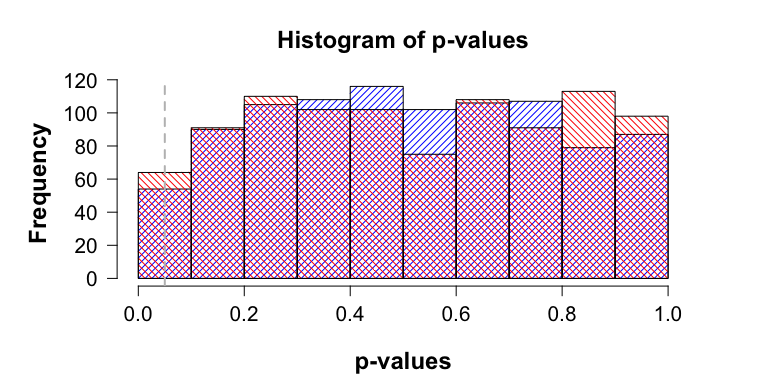The blue histogram represents the distribution of the 954 non-significant p-values calculated over the first batch of data, whereas the red histogram represents the distribution of p-values calculated over the two batches of data combined. The commands pValuesBatch1To2 <- selectivelyContinueDeltaTrueIsZeroWithP$newValues
sum(pValuesBatch1To2 < alpha)
#>  28

show that by extending the non-significant results of the first batch with a second batch of data, we got another 28 false null rejections. This brings the total number of incorrect null rejections to 74 out of 1,000 experiments, hence, 7.4%, which is above the tolerable \alpha-level.

The reason why p-values overreject the null under optional stopping and optional continuation is due to p-values being uniformly distributed under the null. As such, if the null holds true and the number of samples increases, then the p-value meanders between 0 and 1, thus, eventually crossing any fixed \alpha-level.

### Two ways to optionally continue studies with safe tests

Safe tests, as we will show below, do conserve the type I error rate under optional continuation. Optional continuation implies gathering more samples than was planned for because, for instance, (1) more funding came available and the experimenter wants to learn more, (2) the evidence looked promising, (3) a reviewer or editor urged the experimenter to collect more data, or (4) other researchers attempt to replicate the first finding.

A natural way to deal with the first three cases is by computing an e-value over the combined data set. This is permitted if the data come from the same population, and if the e-value used is a test martingale, which the e-values in this package are.

Replication attempts, however, are typically based on samples from a different population. One way to deal with this is by multiplying the e-value computed from the original study with the e-value computed from the replication attempt. In this situation, the e-value formula for the replication study could also be redesigned through the function, for example when more information on nuisance parameters or effect size has become available for designing a more powerful test.

We show that both procedures are safe, that is, they do not lead to the tolerable type I error rate be exceeded, whereas classical p-values once again overreject.

### a. Optional continuation by extending the experiment does not result in safe tests exceeding the tolerable \alpha-level

In this subsection, we show that only continuing studies for which S \leq 1/ \alpha does not lead to an overrejection of the null. This is because the sampling distribution of e-values under the null slowly drifts towards smaller values as the number of samples increases.

Again, we consider the situation in which we only continue studies for which the original e-values did not lead to a null rejection. For the first batch of e-values, we use the simulation study ran in the previous section, and we recall that under optional stopping we get

dataBatch1 <- list(dataGroup1=simResultDeltaTrueIsZero$safeSim$dataGroup1,
dataGroup2=simResultDeltaTrueIsZero$safeSim$dataGroup2)

eValuesBatch1 <- simResultDeltaTrueIsZero$safeSim$eValues
sum(eValuesBatch1 > 1/alpha)
#>  24

thus, 24 false null rejections out of 1,000 experiments.

The follow-up batches of data will be of the same size as the original, thus, n1Plan=63 and n2Plan=63, and will also be generated under the null. The slow drift to lower e-values is visualised by two histograms. The blue histogram represents the sampling distribution of e-values of the original simulation study that did not resulted in a null rejection. The red histogram represents the sampling distribution of e-values computed over the two batches of data combined. To ease visualisation, we plot the histogram of the log e-values; a negative log e-value implies that the e-value is smaller than one, whereas a positive log e-value corresponds to e-values larger than one. For this we run the following code:

selectivelyContinueDeltaTrueIsZero <-
selectivelyContinueTTestCombineData(oldValues=eValuesBatch1,
designObj=designObj,
alternative="greater",
oldData=dataBatch1,
deltaTrue=0,
seed=2, paired=TRUE,
muGlobal=120, sigmaTrue=15,
moreMainText="Batch 1-2")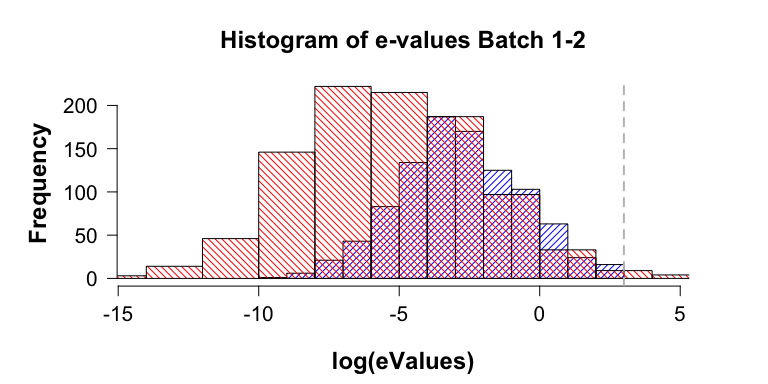Note that compared to blue histogram, the red histogram is shifted to the left, thus, the sampling distribution of e-values computed over the two batches combined concentrates on smaller values. In particular, most of the mass remains under the threshold value of 1/\alpha, which is represented by the vertical grey line \log(1/\alpha) \approx 3.00. This shift to the left is caused by the increase in sample sizes from n1=n2=63 to n1=n2=126. The commands

eValuesBatch1To2 <- selectivelyContinueDeltaTrueIsZero$newValues sum(eValuesBatch1To2 > 1/alpha) #>  7 length(eValuesBatch1To2) #>  976 show that 7 out of the 976 of the selectively continued experiments (0.7%) now result in a null rejection due to optional continuation. Hence, after the second batch of data the total number of total number of false null rejections is 31 out of a total of a 1,000 original experiment, thus, 3.1%. One might wonder whether further extending the non-rejected experiment will cause the total false rejection rate go above 5%. The following code suggests that it does not: for (j in 1:3) { oldEValues <- selectivelyContinueDeltaTrueIsZero$newValues
oldData <- selectivelyContinueDeltaTrueIsZero$combinedData selectivelyContinueDeltaTrueIsZero <- selectivelyContinueTTestCombineData(oldValues=oldEValues, designObj=designObj, alternative="greater", oldData=oldData, deltaTrue=0, seed=2+j, paired=TRUE, muGlobal=120, sigmaTrue=15, moreMainText=paste("Batch: 1 to", j+2)) print(paste("Batch: 1 to", j+2)) print(paste("Number of rejections:", sum(selectivelyContinueDeltaTrueIsZero$newValues > 1/alpha)))
}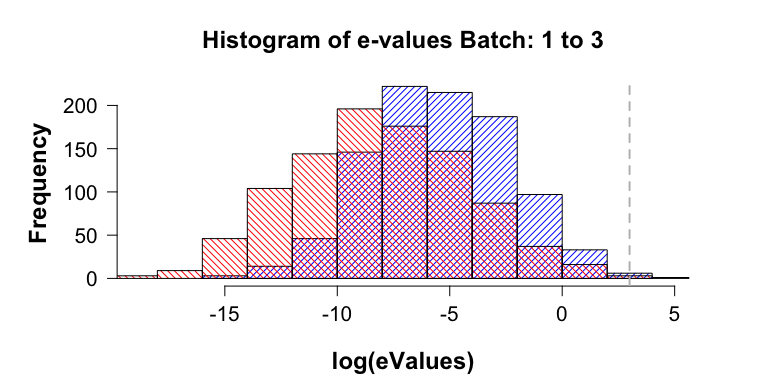#>  "Batch: 1 to 3"
#>  "Number of rejections: 1"
#>  "Batch: 1 to 4"
#>  "Number of rejections: 0"
#> Warning in safeTTestStat(t = t, parameter = designObj[["parameter"]], n1 =
#> n, : Overflow: e-value smaller than 0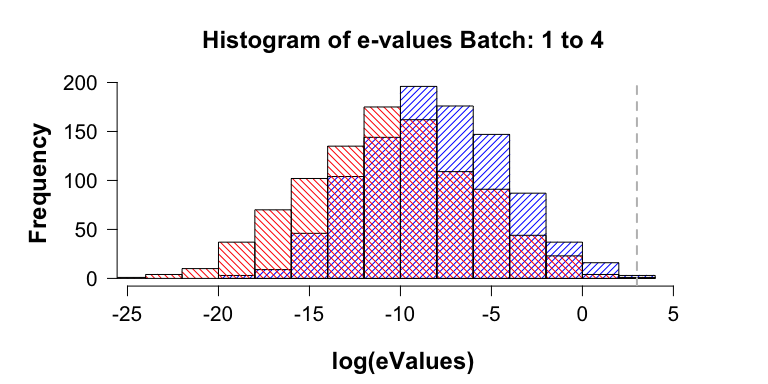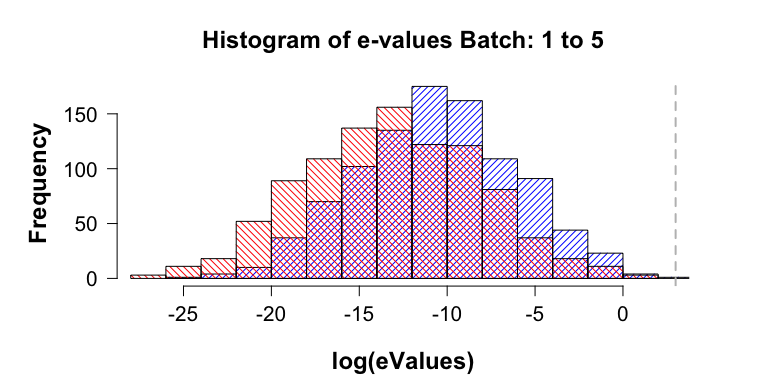#>  "Batch: 1 to 5"
#>  "Number of rejections: 0"

The simulations show that the realised number of false null rejections decreases as the number of replication attempts increases (24, 7, 1, 0, 0, …). Consequently, the collective rate of false null rejections remains well below the tolerable \alpha-level. The histograms slowly drifting to the left show that the chance of seeing an e-value larger than 1/\alpha decreases under the null as the number of samples increases.

#### When the effect is present optional continuation results in safe tests correctly rejecting the null

The slow drift of the sampling distribution of e-values to smaller values is replaced by a fast drift to large values whenever there is an effect. We again consider the situation in which we continue studies for which the first batch of e-values did not lead to a null rejection. The follow-up batch of data will again be of the same sizes, thus, n1Plan=63 and n2Plan=63, and generated under the assumption that deltaTrue equal deltaMin, as in the first batch.

As a first batch of e-values, we use the simulation study ran in the previous section when deltaTrue equals deltaMin, and we recall that under optional stopping we get

dataBatch1 <- list(
dataGroup1=simResultDeltaTrueIsDeltaMin$safeSim$dataGroup1,
dataGroup2=simResultDeltaTrueIsDeltaMin$safeSim$dataGroup2
)

eValuesBatch1 <- simResultDeltaTrueIsDeltaMin$safeSim$eValues
sum(eValuesBatch1 > 1/alpha)
#>  855

855 correct null rejections, since this simulation is based on data generated under alternative with deltaTrue=deltaMin > 0.

The following code selectively continues the 145 experiments which did not lead to a null rejection:

selectivelyContinueDeltaTrueIsDeltaMin <-
selectivelyContinueTTestCombineData(oldValues=eValuesBatch1,
designObj=designObj,
alternative="greater",
oldData=dataBatch1,
deltaTrue=deltaMin,
seed=2, paired=TRUE, muGlobal=120,
sigmaTrue=15)